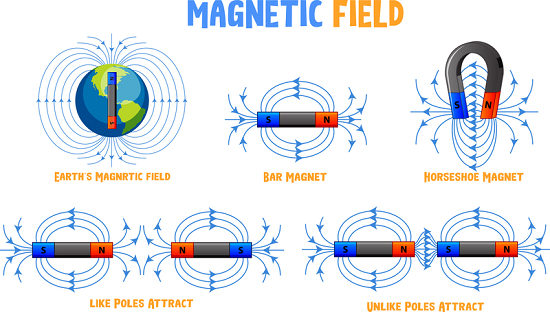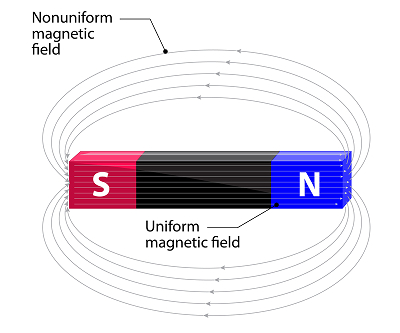# Dipole Uniform Magnetic Field

## Introduction

Whenever a magnet is kept in a magnetic field it suffers force. A magnetic field is a physical field to describe the effect on magnetic materials. The uniformity of the magnetic field has a particular effect on the dipoles in its vicinity. It is very important to learn about the nature of forces and torque acting on the dipole and further understand the reason behind it. On another interesting note, the analogous relation of the magnetic dipole in the magnetic field with the electric dipole in the electric field shall also be derived and pondered upon.## What is a Uniform Magnetic Field?

This is a vector field that explains the magnetic influence and force on any kind of magnetic material or moving charges in its vicinity.A magnetic field can either be created by magnets, moving electric charges or electric currents too. Since it is a vector, it needs both magnitude and direction for its complete description. We often use ‘field lines’ diagrammatically to have an idea about the field generated in space. The field lines representing the magnetic field of a bar magnet are as shown in the image on the side. The closer field lines represent a higher magnitude in that region. The arrow represents the direction of the magnetic field.

A uniform magnetic field just means that the magnitude and the direction of the field are the same throughout the region of our consideration. The magnitude or direction, nothing varies with change in space coordinates. The density of the field lines of a uniform magnetic field in any particular region is going to give us the magnitude of the field.

## What is a Dipole?

A dipole, in very general terms, is the arrangement of the two equal and oppositely charged or magnetized poles by some distance. The arrangement with the electric charges is called an electric dipole, whereas the one with the north and south poles is called a magnetic dipole.

Dipole moment is a physical vector quantity, using which any kind of dipole- whether magnetic or electric can be characterized easily. For an electric dipole, the dipole moment is just the product of the magnitude of the charge and the distance of the separation between them. Whereas the magnetic dipole is a representation of the strength and the orientation of any object that’s producing a magnetic field. A simple bar magnet is an example of a magnetic dipole with a permanent magnetic moment. Even the spinning of a positively charged nucleus in an atom, the revolution of the negatively charged electrons around the nucleus, etc are also examples that make up the magnetic dipole moment.

## The behaviour of Dipole in a Uniform Magnetic Field

Whenever we bring a north pole into a magnetic field (although we can’t bring an isolated magnetic pole), it suffers a force it which is equal to the product of magnetic strength and the value of the magnetic field. Mathematically it can be represented as −

$$\mathrm{F= m\times B}$$

Here m is the pole strength and B is the magnitude of the magnetic field. Whereas, the south pole detects the equal and opposite force. As a result, there is no net force suffered by the dipole. But, as an important point to be kept in mind, both of these forces are acting at distance, say ‘2l’ apart. (The ‘2l’ is taken as the distance of separation of the two poles, we can say the length of the small bar magnet that is kept in a uniform magnetic field B) This results in the net torque in the dipole. Mathematically, it is given by −

$$\mathrm{\tau = force\times distance}$$

$$\mathrm{\tau_{B} = mB\:sin\theta \times 2l}$$

Having 2ml as the Magnetic moment vector ‘M’, we get the formula for torque as

$$\mathrm{\tau_{B} = M\times B}$$

Further, in the calculation of the potential energy of the system,

$$\mathrm{U_{B} = \int \tau _{B}(\theta )d\theta }$$

$$\mathrm{U_{B} = \int MB\:sin \theta \:d\theta }$$

$$\mathrm{U_{B} = - MB\:cos \theta }$$

$$\mathrm{U_{B} = - M.B}$$

## Correlated with Electrostatics

The comparison of the forces, torque and other behavioural effects of the dipole in magneto statics can be done to that in electrostatics. As we have already derived the formula of the torque for a magnetic dipole in a uniform magnetic field, the same can be for an electric dipole in an electric uniform field. The moment m is analogous to p. The pole strength m is analogous to the q charge. further, instead of magnetic field B we have electric field E.

Mathematically, it is given by

$$\mathrm{\tau _{E}=force\times distance}$$

$$\mathrm{\tau _{E}=q\:E\:sin\theta \times 2l}$$

Having 2ql as the Magnetic moment vector ‘p’, we get the formula for torque as

$$\mathrm{\tau _{E}=p\times E}$$

Further, on a calculation of the potential energy of the system,

$$\mathrm{U _{E}=\int T_{E}(\theta )d\theta }$$

$$\mathrm{U _{E}=\int pE\:sin\theta \:d\theta }$$

$$\mathrm{U _{E}=-ME\:cos\theta}$$

$$\mathrm{U _{E}=-M.E}$$

Hence, we see a clear analogy of the electric and magnetic dipoles when kept in a uniform electric field and magnetic field respectively. The negative sign provides us with the convention that the work done against the field (electric or magnetic) increases the potential energy of the system.

## Conclusion

The dipole -both electric and magnetic are in very simple terms arrangements of opposite and equally charged poles separated by some distance. Whenever kept in a uniform magnetic field, despite not suffering any net force, they experience some torque. The expression for the torque and the potential energy derived for that of a magnetic dipole in a uniform magnetic field matches analogously very much to the electric dipole in the uniform electric field. This opens up for us to learn many other beautiful aspects of this analogous relationship between magneto statics and electrostatics.

## FAQs

Q1. What is the nature of the uniform magnetic field lines?

Ans. The uniform magnetic field lines are equidistant parallel lines.

Q2. What do you mean by Lorentz force?

Ans. The net force F on an electric charge q when it enters a region with both electric E and magnetic field B with some velocity v. Mathematically is given by

$$\mathrm{F=q[E+(v\times B)]}$$

Q3. Is magnetic moment a scalar or a vector?

Ans. Magnetic moment is a scalar. Its direction points from south to north of a magnetic material.

For a current-carrying loop, it is in the direction parallel to the plane, according to the right-hand rule-with the fingers curled along the direction of the current flow.

Q4. What is the difference between H and B?

Ans. H is the magnetic field strength which is calculated in units of A/m Whereas, the magnetic flux density B is calculated in Tesla.

Q5. What will be the proportion of the electric force to the magnetic force on an electron that passes undeflected from a region of the uniform field?

Ans. Since the electron goes undeflected in the region, the values of the electric force and magnetic force suffered by it are equal and opposite. Hence, the ratio of both forces is one.

Updated on: 24-Jan-2023

339 Views